# AP Board 5th Class Maths Solutions 4th Lesson Multiplication and Division

Andhra Pradesh AP Board 5th Class Maths Solutions 4th Lesson Multiplication and Division World Textbook Exercise Questions and Answers.

## AP State Syllabus 5th Class Maths Solutions Chapter 4 Multiplication and Division

Mr. Raju is a farmer. He is constructing a new house. He purchased the needed material to build the house like sand. cement, iron, gravel and bricks.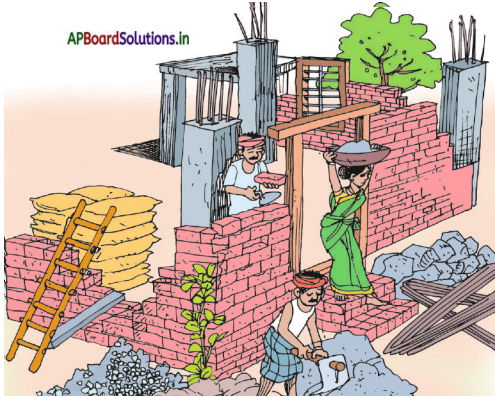The expenses are as mentioned below.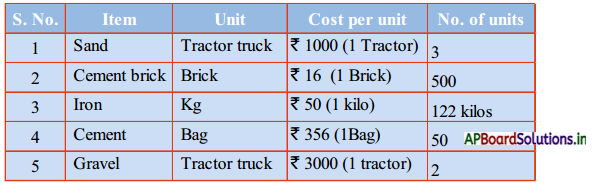How much amount was spent on these primary things?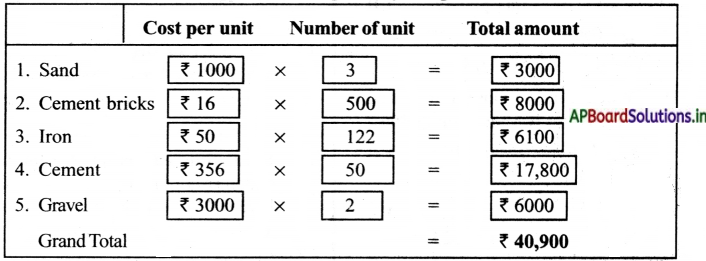Do this: (TextBook Page No.47)

Do the followings:

a) 127 × 12
b) 245 × 17
c) 346 × 19
d) 495 × 24
e) 524 × 36
f) 642 × 43
g) 729 × 56
h) 867 × 69
i) 963 × 72
j) 806 × 83
a) 127 × 12
127 × 2 = 254
127 × 10 = 1270
Total = 1524

b) 245 × 17
245 × 7 = 1715
245 × 10 = 2450
Total = 4,165

e) 346 × 19
346 × 9 = 3114
346 × 10 = 3460
Total = 6,574

d) 495 × 24
495 × 4 = 1980
495 × 20 = 9900
Total = 11,880

e) 524 × 36
524 × 6 =3144
524 × 30 = 15720
Total = 18,864

f) 642 × 43
642 × 3 = 01926
642 × 40 = 25680
Total = 27,606

g) 729 × 56
729 × 50 = 36450
729 × 6 = 4374
Total =40,824

h) 867 × 69
867 × 60 = 52020
867 × 9 7803
Total = 59823

i) 963 × 72
963 × 70 = 67,410
963 × 2 = 1,926
Total = 69,336

j) 806 × 83
806 × 80 = 64480
806 × 3 = 2418
Total = 66898.Do these: (TextBook Page No.58)

1) Do the followings:

a) 2835 × 3
b) 3746 × 5
c) 45392 × 6
d) 56042 × 8
e) 63672 × 9
f) 786435 × 6
g) 79480 × 7
h) 832407 × 6
i) 989235 × 4
j) 905068 × 8
Solution: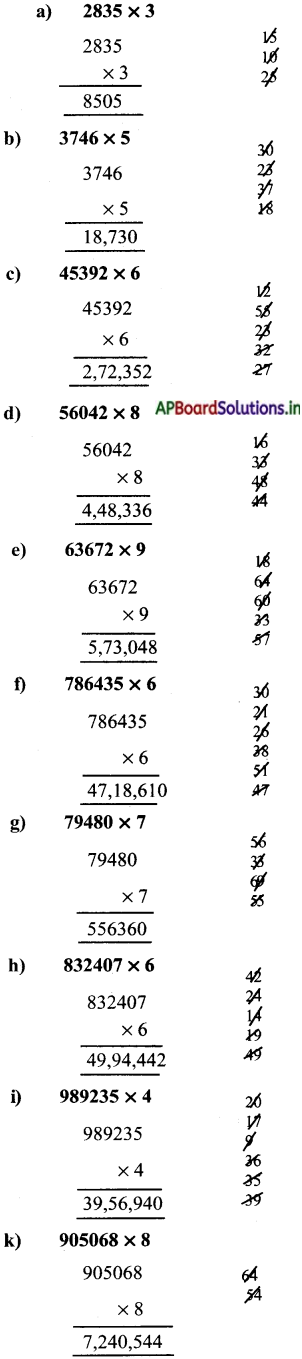Question 2.
A factory manufacturers 4950 cars in a month. How many cars will the factory produce in a year?
Number of cars manufacturers in a month = 4950
Number of ears months in a year = 12
= 4950 × 12
4950 × 10 = 49500
4950 × 2 = 9900
Total = 59,400

Question 3.
If a train travels 143 kilometres in an hour, how far will it travel in one day?
Number of kms travelled by train in an hour = 143
Number of hours per one day = 24 hrs
∴ Distance travelled by train in a day = 143 × 24
143 × 20 = 2860
143 × 4 = 572
Total = 3,432 kms

Do these: (TextBook Page No.50)

1. Do the followings.
a) 3628 × 9
b) 1507 × 69
c) 4256 × 76
d) 27041 × 8
e) 4230 × 121
f) 8271 × 93
a) 3628 × 9
A coffee seller Rohan sells a cup of coffee for ₹ 9. If 3628 cups of coffee was served on a day. How much amount did he earn on that day. How much amount did he earn on that days?

b) 1507 × 69
There are 69 pencils in one box. A shop keeper has 1507 such boxes in his shop. How many pencils does he have in his shop?

c) 4256 × 76
76 roses are needed to make a garland. How many roses are needed to make 4256 such garlands?

d) 27041 × 8
Mrs. Urna maheswari’s monthly salary in 27041. What is her 8 months income?

e) 4230 × 121
There are 121 prisoners in a district jail. How many prisoners are there in 4230 such jails?

f) 827 × 93
There are 93 pages in a book. How many pages are there in 8271 such books?Question 2.
The tea seller Amar sells a cup of tea for ₹ 6. If 1100 cup of teas was served on a day, how much amount did he earn on that day?
Cost of cup of a tea = ₹ 6
Number of cups served = 1100
Amount he earned on that day = 1100 × ₹ 6 = ₹ 6600.

Question 3.
Carpenter Johnson made 9 cots and sold each cot for ₹ 8500. How much amount did he earn?
Cost of each cot = ₹ 8500
Number of cots made by Johnson = 9
Amount he earned = 9 × 8500 = ₹ 76,500

Question 4.
Mr. Kiran works as a scavenger in Nlydukuru municipality. His salary for one month is 18,000. What is his annual salan’ ? Which mathemati cal operation can you use to solve this problem?
Salary for one month = ₹ 18,000
Armual salary of kiran = 1 2 × ₹ 18000 = ₹ 2,16,000
I used commutative property of mathemetical operation.Do this: (TextBook Page No.52)

Question 1.
Find the products:
46 × 23 and 23 × 46
46 × 23 = 1,058
23 × 46 = 1,058

Question 2.
Do the following:
a) 23 × 1 = ____
23

b) 342 × 1 = ____
342

c) 999 × 1 = ____
999

d) 53 × 0 = ____
0

e) 259 × 0 = ____
o

t) 5817 × 0 = ____
0Do this: (TextBook Page No.53)

Estimate the product of these multiplications.

Question 1.
59 × 19
60 × 20 = 1200

Question 2.
99 × 56
100× 60 = 600

Question 3.
189 × 33
200 × 30 =600

Question 4.
4123 × 316
4100 × 300 = 1,230,000Do these: (TextBook Page No.56)

Question 1.
Do the following and write dividend, divisor, quotient and remainder and verify the answer with division relation.
a) 97869 ÷ 6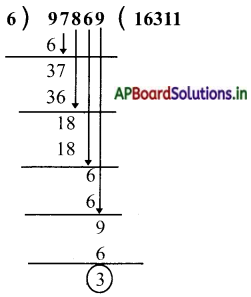Divisor = 6
Dividend = 97869
Quotient= 16311
Remainder = 3
Verification :
Dividend = (Divisor × Quotient) + Remainder
97869 = 6 × 16311 + 3
= 97,866 + 3
97,869 = 97,869

b) 56821 ÷ 9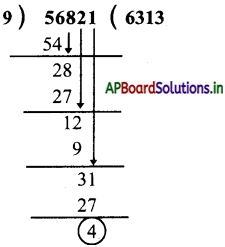Dividend = 56821
Divisor = 9
Quotient = 6313
Remainder = 4
Verification:
Dividend = (Divisor × Quotient) + Remainder
56821 = 9 × 6313 + 4
= 56,817+ 4 = 56,821.c) 68072 ÷ 7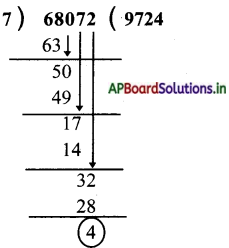Dividend = 68072
Divisor = 7
Quotient = 9724
Remainder 4
Verification :
Dividend = (Divisor × Quotient) + Remainder
68072 = 7 × 9724 + 4
= 68,068 + 4
68,072 = 68,072

d) 10213 ÷ 17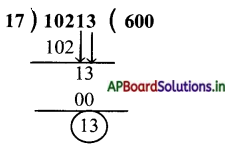Dividend = 10213
Divisor = 17
Quotient = 600
Remainder = 13
Verification:
Dividend = (Divisor × Quotient) + Remainder
10213 = 17 × 600 + 13
= 10200 + 13 = 10213.Question 2.
Raja bought 120 blankets with 6000 to distribute to orphans. What is the cost of each blanket?
Number of blankets bought by Raja = 120
Distributed amount = ₹ 6000
Cost of each blanket = 6000 ÷ 120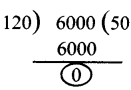∴ Cost of each blanket = 50

Question 3.
Vemaiah bought 100 bread packets to distribute to patients with ₹ 2300. What was the cost of each bread packet?
Number of bread packets distributed by Vemaiah = 100
Distributed amount = ₹ 2300
2300 ÷ 100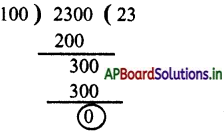∴ Cost of each bread packet = ₹ 23

Try this: (TextBook Page No.56)

Question 1.
Do the following and write your observation.

i) 53427 ÷ 10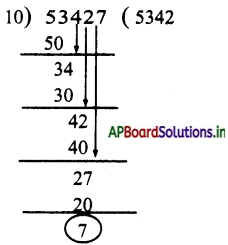ii) 53427 ÷ 100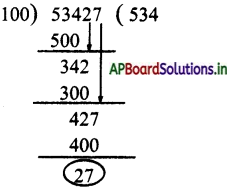iii) 53427 ÷ 1000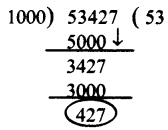iv) 53427 ÷ 10000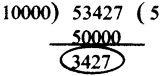Do these: (TextBook Page No.56)

Question 1.
If 8 pots cost is ₹ 800. what is the cost of 5 pots ?
Cost of 8 pots = ₹ 800
Cost of 1 pot = ₹ 800 ÷ 8 = ₹ 1oo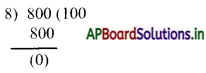∴ Cost of 5 pots = 5 × ₹ 100 = ₹ 500

Question 2.
If 5 kilos tomatoes cost is ₹ 125 what would be the cost of 2 kilos tomatoes?
Cost of 5 kgs tomatoes = ₹ 125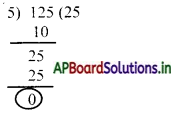Cost of 1 kg tomatoes 25 = ₹ 125 ÷ 5 = ₹ 25
∴ Cost of 2 kgs tomatoes = ₹ 25 × 2 = ₹ 50

Question 3.
A publisher makes 3,875 books in the month of July. If they make the same number of books every day, then how many books can they make in a leap year?
Number of books made by publisher in the month ofJuly = 3,875
Number of days in the month of july = 31
Number of books made by publisher per day = 3875 ÷ 31 = 125 books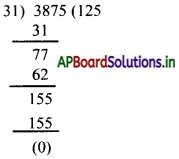Number of days in a leap year = 366
Number of books made by publisher in the leap year = 366 × 125 = 45,750 books.Do this: (TextBook Page No. 58)

Question 1.
Estimate the result.
a) 309 ÷ 10
300 ÷ 10 = 30

b) 497 ÷ 23
500 ÷ 20 = 25

c) 891 ÷ 32
900 ÷ 30 = 30

d) 2940 ÷ 32
3000 ÷ 30 = 100

e) 6121 ÷ 52
6000 ÷ 50 = 120

f) 2928 ÷ 92
3000 ÷ 100 = 30.Question 2.
Jonny bought 5 packets of buns each containing 20 buns to distribute on his birthday. He went to a hospital to distribute the buns. There were 48 patients. Estimate how many buns each patient will get ?
Number of packets brought by Jonny = 5
Number of buns in each packet = 20
Total buns he bought = 5 × 20 = 100
No. of patients in the hospital = 48
Each patient will get = 100 ÷ 50
Approximately = 2 buns

Look at the table and fill in the blanks :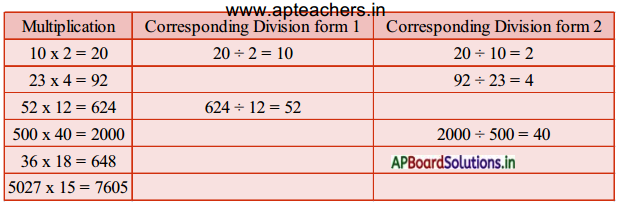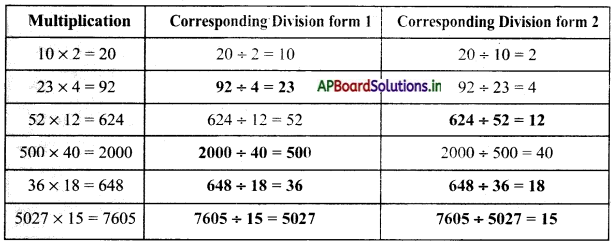Look at the following table and write multiplication – forms for the following divisions.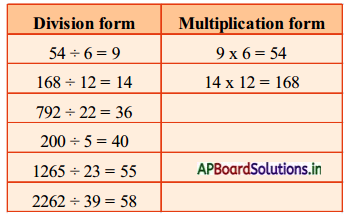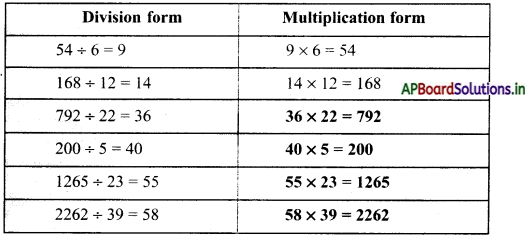Exercise:

Question 1.
Ahmmad earns ₹ 9500 per month. How much amount he earns in a year?
Earnings of Ahmad per month = ₹ 9500
Months per a year = 12
Amount earned by Ahmad per annum = 12 × 9500 = ₹ 114,000

Question 2.
2488 families are living in a major panchayath. If each family pays ₹ 30 per year towards library cess, how much amount will be collected ? Write the process to find the collected amount.
Number of families are living in major Panchayath = 2488
Amount paid by each family per year = ₹ 30
Totally collected amount = 2488 × ₹ 30 = ₹ 74,640

Question 3.
The cost of a bicycle is ₹ 3950. The cost of a motor cycle is 13 times to bicycle’s cost. What is the cost of the motor cycle ?
Cost of a bicycle is = ₹ 3950
Cost of a motor cycle is 13 times to bicycle’s cost
∴ Cost of the motor cycle = 13 × ₹ 3950 = ₹ 51,350.

Question 4.
A carton can hold 36 mangoes. How many such cartons are required if there are 30,744 mangoes in all ?
Total mangoes in all = 30,744
A carton hold in mangoes = 36
∴ Required cartons = 30,744 ÷ 36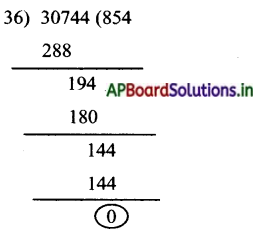∴ Required cartons = 854.Question 5.
Mr. Mani wants to distribute ₹ 64,000 equally among 8 of his workers towards their wages. How much will each worker get ?
Total amount = ₹ 64,000
Number of workers = 8
Amount will each worker get = 64000 ÷ 8 = ₹ 8000

Question 6.
The owner of a cell phone shop bought 8 cell phones of same cost and he gave ₹ 90,000 to wholesaler. The wholesaler returned him ₹ 400. What is the cost of each cell phone ?
Amount given by owner to wholesaler = ₹ 90,000
Amount given by owner to wholesaler = ₹ 90,000 – ₹ 400 = ₹ 89,600
Number of cell phones bought by shop owner = 8
∴ Cost of each cell phone = ₹ 89,600 ÷ 8 = ₹ 11,200

Question 7.
28 laddus weigh 1 kg. How many laddus weigh 12 kgs. If 16 laddus can be packed in one box, how many boxes are needed to pack all these laddus ?
Weight of 28 laddus = 1 kg = 1000 g.
Given weight = 12 kgs
Number of laddus = 12 × 28 = 336
Number of laddus a box conains = 16
Number of boxes required to pack 336 laddus = 336 ÷ 16 = 21 boxes

Question 8.
A fisher man wants to sell 8 kg of fish for ₹ 1600. But Ramu wants to buy 5 kg only. Find the cost for 5 kg.
Cost of a 8 kgs fish = ₹ 1600
Cost of 1 kg fish = 1600 ÷ 8 = ₹ 200
Cost of 5 kg fish = 5 × ₹ 200 = ₹ 1000.Question 9.
50 kgs of jaggery costs ₹ 2500. What is the cost of 15 kg jaggery ?
50 kgs of jaggery cost = ₹ 2500
1 kg of jaggery cost = 2500 ÷ 50
Cost of 15 kgs jaggery = 15 × ₹ 50 = ₹ 750

Question 10.
If a family requires ₹ 3200 for 8 days, how much money does the family require for 4 days ?
Amount required a family for 8 days = ₹ 3200
Amount required a family for 1 day = ₹ 3200 ÷ 8 = ₹ 400
Amount required a family for 4 days = 4 × ₹ 400 = ₹ 1600

Question 11.
Harsha painted pictures and sold them in an art show. He charged ₹ 2567 for big painting. He sold 6 large paintings and 3 small paintings. How much amount did he earn in the art show ?
Charge for big painting = ₹ 2567
Charge for small painting = ₹ 465
Harsha sold 6 large paintings and 3 small paintings.
∴ Amount he earned in the show = 6 × 2567 + 3 × 465 = 15402 + 1395
∴ Total amount = ₹ 16,797

Question 12.
The cost of 63 erasers is ₹ 315. What will be the cost of 42 erasers ?